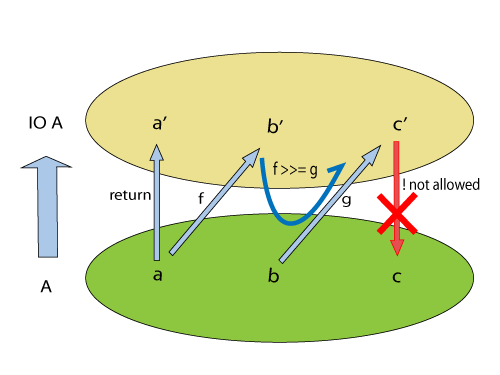## 一. 序章: 可计算模型

1936 阿隆佐·邱奇发表可计算函数的第一份精确定义，即Lambda演算(λ演算)。λ演算是一套从数学逻辑中发展，以变量绑定和替换的规则，来研究函数如何抽象化定义、函数如何被应用以及递归的形式系统。
1945 冯·诺伊曼提出冯·诺伊曼结构(存储程序型电脑)，是通用图灵机实现
1958 约翰·麦卡锡，发明了一种列表处理语言（Lisp），这种语言是一种阿隆佐lambda演算在现实世界的实现，而且它能在冯·诺伊曼计算机上运行

## 三. 函数式编程概念

• 命令式: 代表存储可变状态的单元(内存地址)，相当于地址的别名 x = x + 1
• 函数式: 代表数学函数中的变量，映射到某个值，相当于值的别名 2x = 4

• 命令式: 描述求解过程(怎么做)，本质上是一系列的冯诺依曼机指令，can do anything
• 函数式: 数学概念里的函数，描述映射(计算)关系(做什么)，也称为纯函数/无状态函数

## 四. 函数式编程特性

### 1. 不可变语义

``````// 命令式写法: 直接修改内存值
func (p *Point) moveBy(deltaX, deltaY int) {
p.x = p.x + deltaX
p.y = p.y + deltaX
}

// 函数式写法: 新建一个 Point 对象，函数本身只需关心对象的映射，而非对象复用等实现细节。在语义上，新旧 Point 代表完全独立并可不变的两个"Point 值"。
func moveBy(p *Point, deltaX, deltaY int) *Point {
return &Point{
x:    p.x+deltaX,
y:    p.y+deltaY,
}
}
``````

### 2. 纯函数

• 确定性: 相同输入得到相同输出，函数的返回值和参数以外的其他隐藏信息或状态无关
• 无副作用: 函数不能有语义上可观察的副作用，诸如“触发事件”，使输出设备输出，或更改输出值以外对象的内容等

### 3. 递归与尾递归``````// 自底向上，递推求解
func fact_1(n int) int {
acc := 1
for k:=1; k<=n; k++ {
acc = acc*k
}
return acc
}

//  自顶向下，递归求解
func fact_2(n int) int {
if n == 0 {
return 1
} else {
return fact_2(n-1)*n
}
}

// 自顶向下，递归求解 - Erlang
fact(N) when N == 0 -> 1;
fact(N) when N > 0 -> N*fact(N-1).

// 递归求解 - Erlang - 尾递归版
fact1(N) -> fact(1, N).
fact(Acc, N) when N == 0 -> Acc;
fact(Acc, N) when N > 0 -> fact(Acc*N, N-1).
``````

``````// 若干面值钞票，给一张大面值货币要兑换成零钱，求有多少种兑换方式
// 递归版本
func countChange(money int, coins []int) int {
if money < 0 || len(coins) == 0 {
return 0
}
if money == 0 {
return 1
}
return countChange(money, coins[1:]) + countChange(money-coins, coins)
}

// 迭代版本
func countChange2(changes []int, money int) int {
n  := len(changes)
dp := make([]int, money+1)
for i:=1; i<=money; i++ {
dp[i] = 0
}
dp = 1
for i:=0; i<n; i++ {
for j:=changes[i]; j<=money; j++{
dp[j] += dp[j-changes[i]]
}
}
return dp[money]
}
``````

### 4. 惰性求值/乱序求值

``````A = dosomething1()
B = dosomething2(A)
C = dosomething3()
``````

### 5. 柯里化(currying)

``````fact2(Acc) ->
fun(N) -> fact(Acc, N) end.

F = fact2(1)
F(10)
``````

``````add = function(x, y)
return x + y
end
``````

``````add = function(x)
return function(y)
return x + y
end
end
``````

### 6. 高阶函数

``````sumInts(A, B) when A > B -> 0;
sumInts(A, B) -> A + sumInts(A+1, B).

sumFacts(A, B) when A > B -> 0;
sumFacts(A, B) -> fact1(A) + sumFacts(A+1, B).

sum(F, A, B) when A > B -> 0;
sum(F, A, B) -> F(A) + sum(F, A+1, B).

> sum(fun fact1/1, 3, 6)
``````

``````37> L = [1,2,3].
[1,2,3]
38> lists:map(fun(E) -> 2*E end, L).
[2,4,6]
39> lists:foldl(fun(E, Acc) -> Acc+E end, 0, L).
6
````````````name2reply :: String -> String
"Pleased to meet you, " ++ name ++ ".\n" ++
"Your name contains " ++ charcount ++ " characters."
where charcount = show (length name)

main :: IO ()
main = do
putStrLn "Greetings once again.  What is your name?"
inpStr <- getLine
let outStr = name2reply inpStr
putStrLn outStr
``````

## 五. 函数式的优缺点

• 并发性: 函数无副作用(天然可重入)，原生并发友好
• 确定性: 可读性高，易于测试和调试，错误易于重现
• 没有锁和指针就没有伤害
• 具有很大的优化潜力，如惰性求值，并发，缓存函数计算结果等，很多原本需要程序来做的事情，都可以由编译器来做。比如动态规划的缓存，MapReduce 等。

• 处理可变状态如 IO 的能力弱(要么使用可变状态，要么使用 Monad)
• 为了维持不可变性，拷贝的开销
• 运行效率，依靠并发

## 六. 函数式的实现

### 1. Erlang

Erlang 不是一门纯函数式语言(提供了外部可变状态组件，如进程字典，Ets)，但它充分利用函数式的无状态和不可变语义，将函数式的各种优势很好地利用了起来。Erlang 的具体介绍和细节我们就谈了，我着重讲一下其三个让其它语言”眼红”的三个特性:

#### 高并发

Erlang 是 Actor 模型，一个 Actor 即一个 Erlang 进程，创建一个进程是微秒级的。Erlang 可以说是最早支持协程的语言之一，Actor 与 Actor 之间通过消息交互，加上不可变语义，使得 Erlang 比其它语言更易于实现安全的并发(Erlang/OTP并发编程实践作者将共享内存比作这个时代的goto)。

#### 容错性

Erlang 有一个很有意思的slogan，叫”let it crash”，这看起来与命令式编程中的”defensive coding(防御式编程)”背道而驰，在大型分布式程序的构建过程中，代码会遇到各种各样的异常，代码编写者不可能或者说很难预料并且处理到所有的异常，在这个背景下，Erlang 提出”let it crash” 的概念: 既然未预料的异常无可避免，那么就应该统一隔离处理并且恢复它，注意，这里提到了三个词: 错误隔离，错误处理和故障恢复，大部分语言最多做到第一步(比如 Go 语言)，而 Erlang 把整个三步都做到了虚拟机中。一个进程挂了，会由监督者发现，并且根据重启策略重启。 Actor 的状态隔离与轻量为整个容错性提供基础保障。

• 支持惰性求值
• 更彻底的函数(+,-)
• 静态类型系统 + 类型推导 + 强大的typeclass (==, Functor)，支持自定义类
• 原生柯里化(`map (+1) [1,2,3]`)

## 七. 最后

#### 1. 为什么函数式没有崛起?

• 函数式语言: 正确性 = 一致性 > 完整性 > 简单(为了接口简单，宁愿实现复杂)
• 命令式语言: 简单性（实现简单优于接口简单）> 正确性 > 完整性 > 一致性